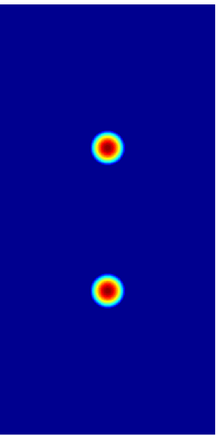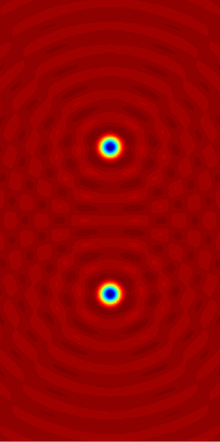# Helmholtz equationTwo sources of radiation in the plane, given mathematically by a function ƒ which is zero in the blue region.The real part of the resulting field A, A is the solution to the inhomogeneous Helmholtz equationIn mathematics, the Helmholtz equation, named for Hermann von Helmholtz, is the partial differential equationwhere 2 is the Laplacian, k is the wavenumber, and A is the amplitude.

## Motivation and uses

The Helmholtz equation often arises in the study of physical problems involving partial differential equations (PDEs) in both space and time. The Helmholtz equation, which represents a time-independent form of the wave equation, results from applying the technique of separation of variables to reduce the complexity of the analysis.

For example, consider the wave equationSeparation of variables begins by assuming that the wave function u(r, t) is in fact separable:Substituting this form into the wave equation, and then simplifying, we obtain the following equation:Notice the expression on the left-hand side depends only on r, whereas the right-hand expression depends only on t. As a result, this equation is valid in the general case if and only if both sides of the equation are equal to a constant value. From this observation, we obtain two equations, one for A(r), the other for T(t):andwhere we have chosen, without loss of generality, the expression −k2 for the value of the constant. (It is equally valid to use any constant k as the separation constant; −k2 is chosen only for convenience in the resulting solutions.)

Rearranging the first equation, we obtain the Helmholtz equation:Likewise, after making the substitutionthe second equation becomeswhere k is the wave vector and ω is the angular frequency.

We now have Helmholtz's equation for the spatial variable r and a second-order ordinary differential equation in time. The solution in time will be a linear combination of sine and cosine functions, with angular frequency of ω, while the form of the solution in space will depend on the boundary conditions. Alternatively, integral transforms, such as the Laplace or Fourier transform, are often used to transform a hyperbolic PDE into a form of the Helmholtz equation.

Because of its relationship to the wave equation, the Helmholtz equation arises in problems in such areas of physics as the study of electromagnetic radiation, seismology, and acoustics.

## Solving the Helmholtz equation using separation of variables

The solution to the spatial Helmholtz equationcan be obtained for simple geometries using separation of variables .

### Vibrating membrane

The two-dimensional analogue of the vibrating string is the vibrating membrane, with the edges clamped to be motionless. The Helmholtz equation was solved for many basic shapes in the 19th century: the rectangular membrane by Siméon Denis Poisson in 1829, the equilateral triangle by Gabriel Lamé in 1852, and the circular membrane by Alfred Clebsch in 1862. The elliptical drumhead was studied by Émile Mathieu, leading to Mathieu's differential equation. The solvable shapes all correspond to shapes whose dynamical billiard table is integrable, that is, not chaotic. When the motion on a correspondingly-shaped billiard table is chaotic, then no closed form solutions to the Helmholtz equation are known. The study of such systems is known as quantum chaos, as the Helmholtz equation and similar equations occur in quantum mechanics (see Schrödinger equation).

If the edges of a shape are straight line segments, then a solution is integrable or knowable in closed-form only if it is expressible as a finite linear combination of plane waves that satisfy the boundary conditions (zero at the boundary, i.e., membrane clamped).

An interesting situation happens with a shape where about half of the solutions are integrable, but the remainder are not. A simple shape where this happens is with the regular hexagon. If the wavepacket describing a quantum billiard ball is made up of only the closed-form solutions, its motion will not be chaotic, but if any amount of non-closed-form solutions are included, the quantum billiard motion becomes chaotic. Another simple shape where this happens is with an "L" shape made by reflecting a square down, then to the right.

If the domain is a circle of radius a, then it is appropriate to introduce polar coordinates r and θ. The Helmholtz equation takes the formWe may impose the boundary condition that A vanish if r = a; thusThe method of separation of variables leads to trial solutions of the formwhere Θ must be periodic of period 2π. This leads toandIt follows from the periodicity condition thatand that n must be an integer. The radial component R has the formwhere the Bessel function Jn(ρ) satisfies Bessel's equationand ρ = kr. The radial function Jn has infinitely many roots for each value of n, denoted by ρm,n. The boundary condition that A vanishes where r = a will be satisfied if the corresponding wavenumbers are given byThe general solution A then takes the form of a doubly infinite sum of terms involving products ofThese solutions are the modes of vibration of a circular drumhead.

### Three-dimensional solutions

In spherical coordinates, the solution is:This solution arises from the spatial solution of the wave equation and diffusion equation. Hereandare the spherical Bessel functions, andare the spherical harmonics (Abramowitz and Stegun, 1964). Note that these forms are general solutions, and require boundary conditions to be specified to be used in any specific case. For infinite exterior domains, a radiation condition may also be required (Sommerfeld, 1949).

Forfunctionhas asymptoticswhere function f is called scattering amplitude andis the value of A at each boundary point.

## Paraxial approximation

In the paraxial approximation of the Helmholtz equation, the complex amplitude A is expressed aswhere u represents the complex-valued amplitude which modulates the sinusoidal plane wave represented by the exponential factor. Then under a suitable assumption, u approximately solveswhereis the transverse part of the Laplacian.

This equation has important applications in the science of optics, where it provides solutions that describe the propagation of electromagnetic waves (light) in the form of either paraboloidal waves or Gaussian beams. Most lasers emit beams that take this form.

The assumption under which the paraxial approximation is valid is that the z derivative of the amplitude function u is a slowly-varying function of z:This condition is equivalent to saying that the angle θ between the wave vector k and the optical axis z is small:.

The paraxial form of the Helmholtz equation is found by substituting the above-stated expression for the complex amplitude into the general form of the Helmholtz equation as follows:Expansion and cancellation yields the following:Because of the paraxial inequalitiy stated above, the ∂2u/∂z2 term is neglected in comparison with the k·∂u/∂z term. This yields the paraxial Helmholtz equation. Substitutingthen gives the paraxial equation for the original complex amplitude A:The Fresnel diffraction integral is an exact solution to the paraxial Helmholtz equation.

There is even a subject named "Helmholtz optics" based on the equation, named in honour of Helmholtz.   

## Inhomogeneous Helmholtz equation

The inhomogeneous Helmholtz equation is the equationwhere ƒ : Rn  C is a given function with compact support, and n = 1, 2, 3. This equation is very similar to the screened Poisson equation, and would be identical if the plus sign (in front of the k term) is switched to a minus sign.

In order to solve this equation uniquely, one needs to specify a boundary condition at infinity, which is typically the Sommerfeld radiation conditionuniformly inwith, where the vertical bars denote the Euclidean norm.

With this condition, the solution to the inhomogeneous Helmholtz equation is the convolution(notice this integral is actually over a finite region, sincehas compact support). Here,is the Green's function of this equation, that is, the solution to the inhomogeneous Helmholtz equation with ƒ equaling the Dirac delta function, so G satisfiesThe expression for the Green's function depends on the dimensionof the space. One hasfor n = 1,for n = 2, whereis a Hankel function, andfor n = 3. Note that we have chosen the boundary condition that the Green's function is an outgoing wave for.

## Notes

1. J. W. Goodman. Introduction to Fourier Optics (2nd ed.). pp. 61–62.
2. Raffaele Grella, Fresnel propagation and diffraction and paraxial wave equation, Journal of Optics 13, 367 (1982)
3. Kurt Bernardo Wolf and Evgenii V. Kurmyshev, Squeezed states in Helmholtz optics, Physical Review A 47, 3365–3370 (1993).
4. Sameen Ahmed Khan, Wavelength-dependent modifications in Helmholtz Optics, International Journal of Theoretical Physics, 44(1), 95http://www.maa.org/programs/maa-awards/writing-awards/can-one-hear-the-shape-of-a-drum125 (January 2005).
5. Sameen Ahmed Khan, A Profile of Hermann von Helmholtz, Optics & Photonics News, Vol. 21, No. 7, pp. 7 (July/August 2010).
6. ftp://ftp.math.ucla.edu/pub/camreport/cam14-71.pdf